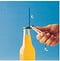# Mechanical advantage Formula of simple machines — PhysicsTeacher.in

In this post, we will talk about the Mechanical advantage formula for different simple machines.

Just to give you a quick heads up, the Mechanical Advantage of a machine is the ratio of output force and the input force.

In other words, it is the ratio of Load and Effort.

Now to understand these in details we need to understand the concepts behind.

I hope you will be with me to go through this topic and understand the minute details.

Before deriving the mechanical advantage formula, let’s see how a machine provides us some kind of advantage and makes our life easier.

# Give examples of simple machines and complex machines.

We use different types of machines in our daily life. They may be:
1> Simple machines — Some machines are simple tools or simple machines like bottle openers, screwdrivers, and scissors.
or,
2> Complex machines — Some machines are complex like bicycles and automobiles.

We understand that Machines make our lives easier. But how?

# Explain Mechanical Advantage of a bottle opener

Consider the bottle opener in Figure 1.fig 1: bottle opener Fe is the input Effort force and Fr is the output resistance force

When we use the opener the following steps are followed:

# Input and Output force, Effort and Resistance force

1) Input force: We lift the handle of the bottle opener. That means we apply an upward input force or Effort force on it. (say Fe as shown in the figure above ) The handle of the bottle opener in our hands experiences an upward displacement. Hence work is done on the opener.

2) Output force: The opener, in turn, transfers a force (output force say Fr as shown in the figure above) on the cap and lifts it. Thus the opener does work on the cap. This output force exerted by the bottle opener or any other simple machine is also known as a Resistance force because it overcomes a Load or a Resistance. In this case, the Load is the cap of the bottle.

# Input and Output Work

3) Input and Output work: Remember, the work that we do is called the input work, Wi. The work that the machine does is called the output work, Wo.
We know that as work is done it causes the transfer of energy by mechanical means.

(a) When we put work into a machine, such as a bottle opener then we transfer energy to the opener.

(b) The opener, in turn, does work on the cap and transfers energy to the cap.

**We must understand that the opener is not a source of energy, and therefore, the opener can’t provide the cap more energy than the amount of energy that we put into the opener.

Thus, the output work can never be greater than the input work.

The machine simply aids in the transfer of energy from us to the bottle cap.

# Formula for Mechanical Advantage

4) Now, what is the relation between this Resistance force(output force) and the Effort force(input force) of a machine?

Here we get the term we are discussing in this post. It is a Mechanical Advantage.

Here we write,

The machine uses this resistance force to lift or pull a load. So resistance force is equal (magnitude wise) to the load overcome by the machine.

Therefore, we can also write
Mechanical Advantage MA = Load / Effort

Q) Write different forms of Mechanical Advantage Formula.

The mechanical advantage, MA, of the machine can be formulated as the

1> The ratio of the output force and the input force
or
2> The ratio of the Resistance Force and the Effort Force
or
3> The ratio of the Load overcome and the applied Effort Force.

# Read our popular topic: Mechanical advantage of a Lever

Originally published at https://physicsteacher.in on September 15, 2019.

NIT Grad & IT prof. Writes and teaches HS physics. A motivational speaker and an active blogger with multiple niches. With lots of stories to tell.

## More from Anupam

NIT Grad & IT prof. Writes and teaches HS physics. A motivational speaker and an active blogger with multiple niches. With lots of stories to tell.